Metonymy and Metaphor in Mathematics

## Metaphors from modern mathematics: I. Fourier analysis and the dot-product

Metonymy is everywhere in mathematics, but a good metaphor is hard to find. This one and the next are two of my favorites.

In the simplest form of Fourier analysis, the calculation of the Fourier sine series for an odd function f(x) defined on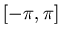, the function is represented as a linear combination of the functionsetc.where the coefficients b1, b2, etc. are given by the integrals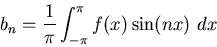The metaphier. In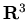, a vector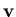can be represented as a linear combination of the basis elements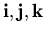: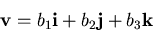where the coefficients b1, b2, etc. are given by the dot products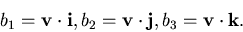This works because the basisis orthonormal: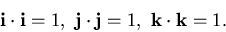The metaphor: in the vector space of piecewise-smooth, odd functions on, define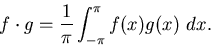Then the functions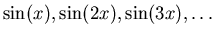are orthonormal with respect to this dot-product, as can be checked by carrying out the integrals. So writing the Fourier sine series for f is exactly analogous to writing v as a linear combination ofusing the dot-products as coefficients.

(For more details see a text like David Powers, Boundary Value Problems Academic Press, New York 1972 or Walter Rudin, Principles of Mathematical Analysis McGraw Hill, New York 1974.)